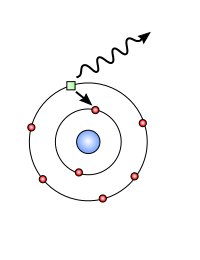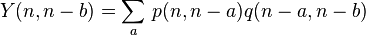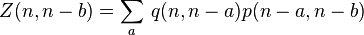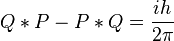# Conjugate variables facts for kids

Kids Encyclopedia Facts

Conjugate variables are special pairs of variables (like x, y, z) that don't give the same result when you do a certain mathematical operation with them. This means that x*y is not equal to y*x. Here, the * does not mean multiplication. It could mean addition, subtraction, division, or any operation that makes sense, in that case.

A physicist, Werner Heisenberg, and his co-workers used equations studied in classical physics to describe and predict events from quantum physics. He discovered that the momentum (mass times velocity, represented by P) and position (represented by Q) are conjugate variables. This means that P*Q is not equal to Q*P, in quantum physics.

Here are two special equations to calculate the energy of an electron (small green thing) in a hydrogen atom.The first equation could be used to find out the product of momentum and position:$Y(n,n-b) = \sum_{a}^{} \, p(n,n-a)q(n-a,n-b)$

The second equation could be used to calculate the product of position and momentum:$Z(n,n-b) = \sum_{a}^{} \, q(n,n-a)p(n-a,n-b)$

Some time later, another physicist, Max Born found out that, because P*Q is not equal to Q*P, the result of Q*P minus P*Q is not zero. (The "minus" is not the same minus of "3 - 2". It's a different thing with the same name).

Born found out that:${Q*P - P*Q = \frac{ih}{2\pi}}$

[The symbol Q is the matrix for position, P is the matrix for momentum, i is a complex number, and h is Planck's constant, a number that shows up in quantum mechanics a lot.]

Conjugate variables have applications all over Physics, in Chemistry and in a bunch of other areas of science.

## Some related topicsConjugate variables Facts for Kids. Kiddle Encyclopedia.